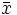Clifford algebras were invented to understand rigid transformations on their base space V. To explore the zero divisors of C we consider the action on C of multiplying on the left by an element x of C. In particular consider the action of multiplying by an element of {x| m(x)=1} where “m(x)” = |x|2 = <x> = (mag2 x) = (sum of squares of scalar coefficients in basis expansion of x) . λx.m(x) = m is not one of the functions normally of significance in Clifford algebras but it is a coordinate free function. Some of these formulae fail if V’s quadratic form is not positive definite.

m(xy) = m(x)m(y) is true for octonions but not for sedenions. Octonions are a divisions algebra and sedenions are not even though most sedenions have inverses. m(xy) = m(x)m(y) is true for Cl(n, 0) only if 0≤n<3.

C = Cl(4, 0),
V is the 4D space within C that generates C,
BV = {γ0, γ1, γ2, γ3} is an orthonormal basis for V,
t = γ0γ1γ2γ3,
For set x, P(x) is the power set of x, sometimes written 2x. It is the set of subsets of x.
If x ⊆ C and x is finite then Π(x) is the product of the elements of x. Π(∅) = 1. Π(BV) = t. (Π is ambiguous due to lack of commutativity but I use it where the only ambiguity is the sign and where sign doesn't matter.)
If x ⊆ C then −x = {−z | z ∊ x}.
BC = {Π(x) | x⊆BV} is a basis for C.

The code below reveals that the set B = {a(t+1) | a ∊ C} is an 8 dimensional subspace of left zero divisors which is spanned by a set Q of 8 independent Clifford numbers. Q = {1+γ0123, γ0123, γ1−γ023, γ01−γ23, γ2013, γ0213, γ12−γ03, γ012−γ3}.

The code works as follows: Q = {x(t+1)|x ∊ BC}. Q spans B.

B is closed under multiplication. An example of aa=0: (γ0123)(γ0123) = 0.

The code below uses definitions from here.
```; generate set of subsets where set is a list:
(define (P a) (if (null? a) '(()) (let ((c (car a))) (let L ((x (P (cdr a))))
(if (null? x) '() (cons (car x) (cons (cons c (car x)) (L (cdr x)))))))))

; multi operand multiply:
(define (lm x)(if (null? x) C1 (C* (car x) (lm (cdr x)))))

; generate all subsets of basis for V then generate basis for C.
(define CB (map lm (map reverse (P (reverse basis)))))

; numlist turns a number tree into a list of numbers.
; below a must be a number tree and b a number list. Returns a number list.
(define (numlist a) (let nl ((a a)(b '())) (if (number? a) (cons a b)
(nl (car a) (nl (cdr a) b)))))

; observe generation of basis for C suitable for rank software.
; (map numlist (map lm (map reverse (P basis)))) ; => twist permutation.

(define Q (map numlist
(let ((T (C+ C1 (C* (C* g0 g1) (C* g2 g3)))))
(map (lambda (p) (C* p T)) (map lm (map reverse (P basis)))))))

Q => (
(1  0 0  0  0 0  0 0 0  0 0  0  0 0  0 1)
(0  0 0  0  0 0  0 1 1  0 0  0  0 0  0 0)
(0  0 0  0  1 0  0 0 0  0 0 -1  0 0  0 0)
(0  0 0 -1  0 0  0 0 0  0 0  0  1 0  0 0)
(0  0 1  0  0 0  0 0 0  0 0  0  0 1  0 0)
(0  0 0  0  0 1  0 0 0  0 1  0  0 0  0 0)
(0  0 0  0  0 0  1 0 0 -1 0  0  0 0  0 0)
(0 -1 0  0  0 0  0 0 0  0 0  0  0 0  1 0)
(0  1 0  0  0 0  0 0 0  0 0  0  0 0 -1 0)
(0  0 0  0  0 0 -1 0 0  1 0  0  0 0  0 0)
(0  0 0  0  0 1  0 0 0  0 1  0  0 0  0 0)
(0  0 1  0  0 0  0 0 0  0 0  0  0 1  0 0)
(0  0 0  1  0 0  0 0 0  0 0  0 -1 0  0 0)
(0  0 0  0 -1 0  0 0 0  0 0  1  0 0  0 0)
(0  0 0  0  0 0  0 1 1  0 0  0  0 0  0 0)
(1  0 0  0  0 0  0 0 0  0 0  0  0 0  0 1))
which is of rank 8.
The first 8 are independent and for i = 0 thru 7
(15-i)th vector is same or negative of ith vector.
```

Now include the definitions of trnxx and transpose and matpak, ops and inv and Rank.
```
(define (treestr x) (let ld ((x x)) (if (and (pair? x)(null? (cdr x))) (car x)
(ld (let z ((x x)) (if (and (pair? x)(pair? (cdr x)))
(cons (cons (car x)(cadr x)) (z (cddr x))) '()))))))

(define trn transpose)
; The following computes the smallest subset of Q that spans what Q does.
(define Q (map treestr (trn (Rank (trn Q)))))
; Q is the computed set referred to above and (Sr Q) generates random elements form B.
```
Here we automate this so we can perform this process on other discovered zero divisors.